The Mathematical Intelligencer

, Volume 41, Issue 4, pp 1–7

# Counting Parallel Segments: New Variants of Pick’s Area Theorem

Open Access
Article

Pick’s area theorem provides a simple and unexpected formula for calculating the area of simple polygons whose vertices lie on lattice points. Consider the standard square lattice $${\mathbb{Z}}^2$$ consisting of points with integer coordinates. A simple lattice polygon P is the region bounded by a simple (i.e., nonintersecting) closed polygonal chain whose vertices belong to $${\mathbb{Z}}^2$$ (in other words, the vertices have integer coordinates). It is convenient for us to consider the boundary of P to be part of the polygon.

Pick’s area theorem , a famous result in elementary plane geometry, states that the area of a simple lattice polygon P containing B lattice points on its boundary and I lattice points in its interior is given by
$$A(P)=I+\frac{B}{2}-1.$$
(1)
This formula is so simple, elegant, and unexpected that it is routinely included in various collections of elementary and nonelementary mathematical results [11, 2, 1]. Generalizations of (1) [13, 14, 6, 10] have led to a number of interesting mathematical results in the fields of algebraic, discrete, and computational geometry [9, 7, 3, 5] (see also references therein).

A common approach to proving Pick’s area theorem consists in subdividing the polygon P into elementary parts for which (1) can be easily verified. Then an invocation of the additive property of the right-hand side of (1) (formally, $$I+B/2-1$$ is a valuation on the set of lattice polygons ) proves the theorem.

Formula (1) is not valid for general lattice polygons, those that may have holes or whose boundaries may have self-intersections. Following [13, 17, 15], we deal with nonsimple lattice polygons. For a nonsimple lattice polygon P we assume that (a) the boundary of P, $$\partial P$$, may consist of several disconnected components; (b) if two edges of $$\partial P$$ intersect, the intersection point is a lattice point; (c) every boundary segment separates the interior of P from the exterior of P. Figure 1 presents examples of nonsimple lattice polygons.Figure 1Pick’s formula (1) fails for these two lattice polygons. Formula (2) computes the areas correctly. (a) $$I=2$$, $$B=18$$, $$\chi (P)=0$$, $$\chi (\partial P)=0$$, $$A(P)=11$$. (b) $$I=1$$, $$B=13$$, $$\chi (P)=1$$, $$\chi (\partial P)=-1$$, $$A(P)=6.$$
For nonsimple lattice polygons, the following extension of (1) was established by Reeve :
$$A(P)=I+\frac{B}{2}-\chi \left( P\right) +\frac{\chi \left( \partial P\right) }{2},$$
(2)
where $$\chi (P)$$ and $$\chi (\partial P)$$ are the Euler characteristics of P and $$\partial P$$. Figure 1 also presents examples of area calculations using (2).

An elegant result like (1) deserves an elegant proof, and one such proof for Pick’s area theorem was proposed in . The idea is to assume that at time 0, a unit of heat is concentrated at each lattice point. Then at time $$\infty$$, heat is uniformly distributed over the whole plane, and a simple analysis shows that the amount of heat contained within polygon P is given by the right-hand side of (1).

In the following, abusing terminology, the term polygon will mean a nonsimple lattice polygon.

Our approach is inspired by the heat conduction proof of . However, instead of units of heat concentrated at the lattice points, we deal with a family of parallel lattice segments (e.g., the vertical lattice segments). A lattice segment is the segment connecting two lattice points belonging to the lattice graph and containing no other lattice points. We assume that each lattice segment situated, possibly without its endpoints, in the interior of P, a whole segment, contains a unit amount of heat.

Then we expect that each boundary segment, a lattice segment belonging to $$\partial P$$, contains half of the unit amount of heat. It remains to estimate the contribution of the remaining incomplete lattice segments situated in the interior of P. We call a lattice segment partial if it crosses $$\partial P$$ and its intersection with the interior of P is not empty. Our formula for the area of P includes the numbers of whole, boundary, and partial segments. Namely, let w, b, and p denote the numbers of whole, boundary, and partial segments. We have the following result.

### Theorem 1.

The area of a lattice polygon via parallel lattice segments is given by the following formula:
$$A(P)=w+\frac{p}{2}+\frac{b}{2}.$$
(3)
Figures 2 and 3 illustrate this formula. The whole segments are solid blue, partial segments are striped blue, and boundary segments are red. Figure 3 suggests that (3) works with any family of parallel lattice segments, not only with vertical or horizontal segments.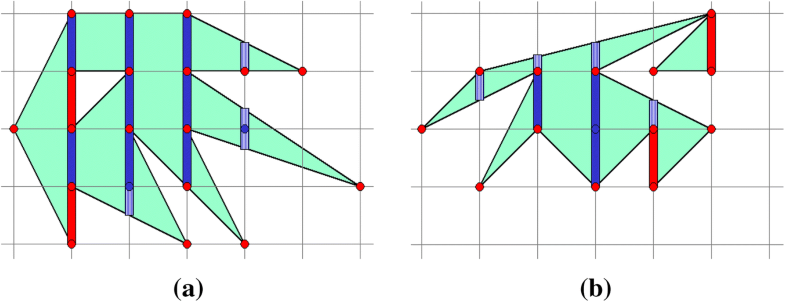Figure 2Illustration of the line segments formula (3). (a) $$w=8$$, $$p=4$$, $$b=2$$, $$A(P)=w+p/2+b/2=11$$. (b) $$w=3$$, $$p=4$$, $$b=2$$, $$A(P)=w+p/2+b/2=6.$$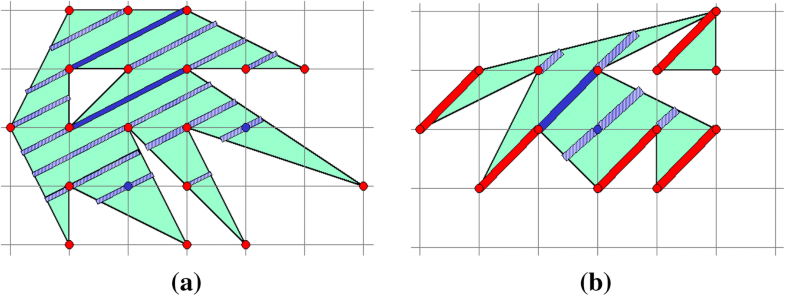Figure 3Illustration of the line segments formula (3) with different families of parallel lattice segments. (a) $$w=2$$, $$p=18$$, $$b=0$$, $$A(P)=w+p/2+b/2=11$$. (b) $$w=1$$, $$p=5$$, $$b=5$$, $$A(P)=w+p/2+b/2=6.$$
Our proof of (3) is straightforward. Let us define a function
$$a(P)=w+\frac{p}{2}+\frac{b}{2}.$$
(4)
Consider a primitive lattice triangle (a lattice triangle is primitive if its three vertices are its only lattice points). It is well known that the area of every primitive lattice triangle is 1/2 (see, for example, [11, Chapter 2]). For a primitive lattice triangle, (4) yields 1/2 (see the left-hand image of Figure 4, for example). We say that a lattice polygon P is decomposed into two lattice polygons $$P_1$$ and $$P_2$$ if $$P=P_1\cup P_2$$ and the interiors of $$P_1$$ and $$P_2$$ have empty intersection. Then
$$a(P)=a(P_1)+a(P_2),$$
which means that a(P) is a valuation. This completes the proof of (3). Figure 4 illustrates the proof visually.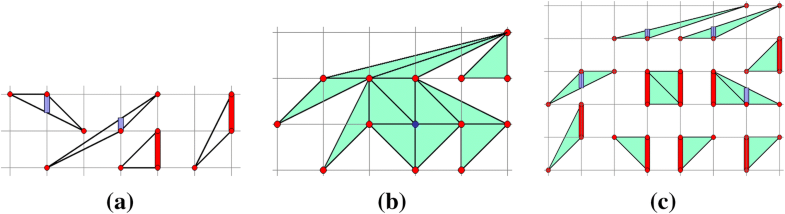Figure 4(a) examples of primitive lattice triangles; for each of them, the function (4) gives 1/2. (b) decomposition of a lattice polygon into primitive lattice triangles. (c) A partial cut of the same lattice polygon.
As demonstrated in Figure 5, like Reeve’s formula (2), our formula (3) can be easily extended to nonsquare lattices.Figure 5Let the area of the fundamental lattice triangle be 1/2. For a given triangle lattice polygon P, we have $$A(P)=13/2=6.5$$. (a) $$I=1$$, $$B=12$$, $$\chi (P)=0$$, $$\chi (\partial P)=-1$$, and (2) gives $$I+B/2-\chi (P)+\chi (\partial P)/2=6.5$$. (b) $$w=4$$, $$p=4$$, $$b=1$$, and our formula (3) yields the same result $$w+p/2+b/2=6.5.$$
Counting radial segments. One can consider radial segments instead of parallel ones, as seen in Figure 6. Given a lattice polygon, let us consider a lattice point and draw a ray passing through the point and each lattice point of the polygon.
• If the origin of the rays is located outside the polygon, as shown in Figure 6(a), then we count the intersection segments in exactly the same way as when the segments are parallel.

• If the origin of the rays is situated in the interior of the polygon, we think of the origin as being excluded from the polygon.

So each ray segment belonging to the polygon and connecting the origin to a polygon lattice point and containing no other lattice points is considered a partial segment, which contributes only 1/2 to the area of the polygon. Similarly, each ray segment connecting the origin to a lattice point outside the polygon and containing no other lattice points is excluded from the area computation. See Figure 6(b) for examples.

• Finally, if the origin is a boundary vertex of the polygon, we also think of it as being excluded from the polygon, and in addition, we assign only 1/4 to a boundary lattice segment connecting the origin to its neighboring boundary vertex, as demonstrated in Figure 6(c) (alternatively, we could introduce partial boundary segments, each of which contributes 1/4 to the area).

It is easy to see that the proposed way of counting the radial segment contributions satisfies the additive property (so it is a valuation) and gives the area if the polygon is a primitive lattice triangle. Thus it yields the area of a general lattice polygon.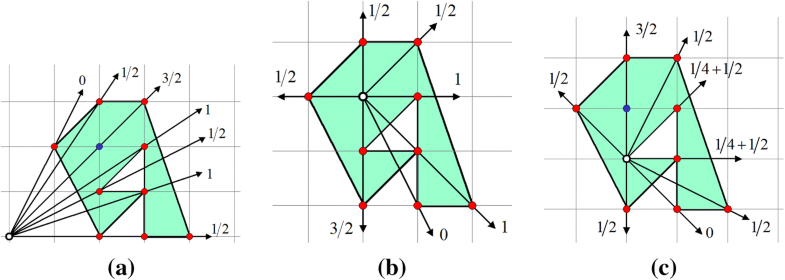Figure 6Counting radial segments. (a) If the origin of the rays is located outside the polygon, we count the intersection segments in exactly the same way as when the segments are parallel. (b) If the origin of the rays is situated in the interior of the polygon, we think of the origin as being excluded from the polygon. (c) If the origin is a boundary vertex of the polygon, we also think of it as being excluded from the polygon. See the text for details.

One can observe that if a simple lattice polygon is star-shaped with respect to a ray-emitting origin situated at an internal lattice point of the polygon, then our approach reproduces Pick’s original formula (1).

## The 3D Case

We deal now with the three-dimensional case of lattice polyhedra. Let us define a polyhedron as a bounded region in 3D space whose boundary consists of a finite number of polygonal faces, each pair of which either are disjoint or have a common edge or common single vertex. In addition, we consider polyhedra without self-crossings and assume that each boundary point of a polyhedron has a surface neighborhood homeomorphic to an open disk. A lattice polyhedron is a polyhedron whose vertices are situated on the cubic lattice $${\mathbb{Z}}^3$$ (thus the vertex coordinates are integers). As in the 2D case, the boundary of a lattice polyhedron P is considered a part of P. We also assume that the polyhedra we deal with can be decomposed into primitive tetrahedra, where a primitive tetrahedron [8, Theorem 4.2] is a tetrahedron in $${\mathbb{R}}^3$$ that does not contain any lattice points in its interior or on its boundary other than its vertices (lattice points are the points of the lattice $${\mathbb{Z}}^3$$).

Extending Pick-type formulas to 3D lattice polyhedra is not easy. First of all, there are polyhedra that cannot be decomposed into tetrahedra. Second, the following simple counterexample demonstrates that there is no simple analogue of Pick’s theorem for polyhedra in 3D . Consider the so-called Reeve tetrahedron, whose vertices have coordinates (0, 0, 0), (1, 0, 0), (0, 1, 0), and (1, 1, r), where r is a positive integer (see Figure 7 for examples of Reeve tetrahedra for $$r=2,3,4$$). The tetrahedron has volume equal to r/6 but contains no lattice points in its interior, and the only boundary lattice points are its vertices.Figure 7(a) to (c) Reeve tetrahedra for $$r=2,3,4$$. (d) The four tetrahedra together. The lines correspond to the edges of the lattice.
Reeve [13, 14] suggested a clever way to generalize Pick’s theorem for polyhedra. Given a lattice polyhedron P, his idea is to consider a sublattice $${\mathbb{Z}}^3/n$$, where n is a positive integer, and construct a formula that takes into account boundary and interior vertices of P in both the original lattice $${\mathbb{Z}}^3$$ and the sublattice. Equivalently, one can consider an inflated polyhedron nP and take into account the numbers of interior and boundary vertices for both the original polyhedron P and its inflated version nP. The resulting formula for the volume $${\rm Vol}(P)$$ is given by
$$2(n-1)n(n+1){\rm Vol}(P)=(2I_n+B_n)-n(2I+B),$$
(5)
where I (respectively $$I_n$$) is the number of lattice vertices in the interior of P (respectively nP) and B ($$B_n$$) is the number of boundary lattice vertices of P (nP) [13, 15]. A variety of similar formulas can be obtained if several sublattices, or equivalently, several inflated versions of P, are simultaneously considered .
Similar to Pick’s theorem, our segment-based approach can be extended to three dimensions if in addition to the original polyhedron P, we consider its inflated version. Namely, let us denote by $$w_{\rm I}$$ the number of whole vertical lattice segments contained, possibly without their endpoints, in the interior of P (inner segments), by $$p_{\rm I}$$ the number of partial vertical lattice segments in the interior of P, by $$w_{\rm B}$$ the number of whole vertical lattice segments contained by $$\partial P$$, and by $$p_{\rm B}$$ the number of partial vertical lattice segments contained by $$\partial P$$. Here a vertical lattice segment is called a partial interior/boundary segment if it belongs to the lattice, contains no other lattice points except its endpoints, and one of its endpoints belongs to the interior/boundary of P, while another endpoint is situated outside of P. See Figure 8 for a visual explanation of these different types of segments.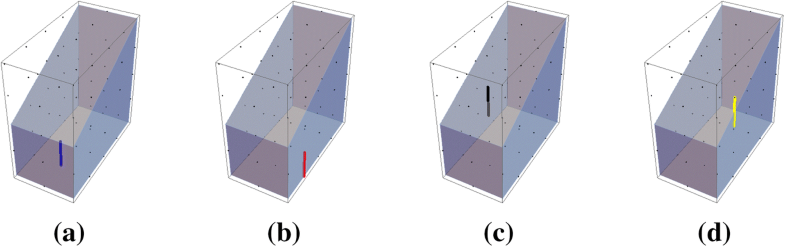Figure 8The different types of lattice segments: (a) whole (blue), (b) boundary whole (red), (c) partial (black), (d) boundary partial (yellow). The lattice points appear as black dots.
Similar to (4), we define
$$v(P)=w_{\rm I}+\frac{p_{\rm I}}{2}+\frac{w_{\rm B}}{2}+\frac{p_{\rm B}}{4}.$$
(6)
Now our formula for the volume $${\rm Vol}(P)$$ of a lattice polyhedron P is given by
$${\rm Vol}(P)=\frac{v(2P)-2v(P)}{6}.$$
(7)

Similar to the 2D case, let us say that a lattice polyhedron P is decomposed into two lattice polyhedra $$P_1$$ and $$P_2$$ if $$P=P_1\cup P_2$$ and the interiors of $$P_1$$ and $$P_2$$ have empty intersection.

If we decompose a given lattice polyhedron P into two lattice polyhedra $$P_1$$ and $$P_2$$, then we have
\begin{aligned} v(P)&=v(P_1)+v(P_2) ,\\ v(2P)&=v(2P_1)+v(2P_2). \end{aligned}
Thus (6) is an additive function on the lattice polyhedra (a lattice valuation) and so is the right-hand side of (7). So it remains to verify that (7) is true for the primitive tetrahedra.
A rigorous verification of (7) on the primitive tetrahedra would likely be tedious. Instead, we use the approach proposed by Reeve [13, 14] and further developed by Ehrhart . Consider the dilated lattice polyhedron nP, where n is a positive integer. We claim that
$$v(nP)={\rm Vol}(P)n^3+c(P)n,$$
(8)
where c(P) is a coefficient that depends on the polyhedron P. First, let us show that the leading coefficient of v(nP) is $${\rm Vol}(P)$$. Indeed, $$w_{\rm B}(nP)$$, $$p_{\rm B}(nP)$$, and $$p_{\rm I}(nP)$$ are $$O(n^2)$$. Each lattice point in the interior of nP contributes to two vertical segments for which the vertex is an endpoint. So the difference between $$I_n$$, the total number of lattice points in the interior of nP, and $$w_{\rm I}(nP)$$ is also $$O(n^2)$$. On the other hand, (5) implies that $$I_n={\rm Vol}(P)n^3+O(n^2)$$. Thus $$w_{\rm I}(nP)={\rm Vol}(P)n^3+O(n^2)$$. Second, one can see that v(nP) is an odd function with respect to n:
$$v(-nP)=-v(nP)$$
if we deal with directed vertical segments (assume that the positive direction is given by the positive direction of the z-axis). This leads us to (8).

Now (8) implies (7) and suggests also the following more general result.

### Conjecture

(Lattice polyhedron volume via parallel lattice segments). We conjecture that
$${\rm Vol}(P)=\left[ v(nP)-n\,v(P)\right] /(n^3-n),$$
(9)
wherePis a lattice polyhedron, v(P) is defined by (6), and$$n>1$$ is an integer.

We formulate it as a conjecture, since our derivation of (8) and (9) (and its particular case (7)) is not complete: we don’t have a proof that v(nP) is a polynomial in n.

Below we present results of our calculation of (8) for several polyhedra:

Unit cube: $$v(nP)=n^3+n$$.

Reeve tetrahedron ($$r=2$$): $$v(nP)=1/3 n^3 - 1/3 n$$.

Hexahedron from Figure 9: $$v(nP)=3 n^3 + 5/2 n$$.

Toroidal polyhedron from Figure 10: $$v(nP)= 24n^3$$.

The geometric meaning of the coefficient c(P) in (8) remains unclear to us.Figure 9(a) A hexahedron with vertex coordinates (0, 0, 0), (1, 0, 0), (1, 2, 0), (0, 2, 0), (0, 0, 1), (1, 0, 1), (1, 2, 2), (0, 2, 2). (b) The same hexahedron with its 2-inflated version. The black points are the lattice points. (c) The different types of lattice segments (whole or partial, boundary or not) are shown on the 2-inflated version. For this example, $$w^{_{(1)}}_{\rm I}=0$$, $$p^{_{(1)}}_{\rm I}=2$$, $$w^{_{(1)}}_{\rm B}=8$$, $$p^{_{(1)}}_{\rm B}=2$$ and $$w^{_{(2)}}_{\rm I}=8$$, $$p^{_{(2)}}_{\rm I}=6$$, $$w^{_{(2)}}_{\rm B}=34$$, $$p^{_{(2)}}_{\rm B}=4$$, giving a value of 3 for the formula (7), which matches the hexahedron’s volume.Figure 10(a) a toroidal polyhedron with vertex coordinates (1, 1, 0), (1, 1, 2), (3, 1, 0), (3, 1, 2), (3, 3, 0), (3, 3, 2), (1, 3, 0), (1, 3, 2), (0, 0, 0), (0, 0, 2), (4, 0, 0), (4, 0, 2), (4, 4, 0), (4, 4, 2), (0, 4, 0), (0, 4, 2). (b) The polyhedron and its 2-inflated version. For this example: $$w^{_{(1)}}_{\rm I}=0$$, $$p^{_{(1)}}_{\rm I}=0$$, $$w^{_{(1)}}_{\rm B}=48$$, $$p^{_{(1)}}_{\rm B}=0$$ and $$w^{_{(2)}}_{\rm I}=96$$, $$p^{_{(2)}}_{\rm I}=0$$, $$w^{_{(2)}}_{\rm B}=192$$, $$p^{_{(2)}}_{\rm B}=0$$, giving a value of 24 for the formula (7) and matching the polyhedron’s volume.

While (6) was used with vertical lattice segments in the examples in Figures 810, as in the 2D case, (6) can be used with any family of parallel lattice segments (not only vertical ones).

## Final Remarks

Our main contribution in this paper was the use of parallel lattice segments for calculating the area of lattice polygons and the volume of 3D lattice polyhedra. These result in formulas (3) and (9).

Although (7) and (9) look simple and elegant, more formulas can be derived from (8) if, for example, several dilated versions of the original polyhedron P are considered. It would be interesting to see how our results follow from , where a general study of valuations of lattice polygons and polytopes is given.

We have not presented any practical applications of our approach in this paper (obviously there are much more efficient ways to compute the area of a polygon and the volume of a polyhedron ). On the other hand, we hope that just as Pick’s area theorem led to a number of interesting mathematical results [3, 5], our approach may inspire some new developments in discrete and computational geometry.

## References

1. 
M. Aigner and G. M. Ziegler. Proofs from the BOOK, 6th edition. Springer, 2018.Google Scholar
2. 
C. Alsina and R. B. Nelsen. Charming Proofs: A Journey into Elegant Mathematics. MAA, 2010.Google Scholar
3. 
M. Beck and S. Robins. Computing the Continuous Discretely. Springer, 2007.Google Scholar
4. 
C. Blatter. Another proof of Pick’s area theorem. Mathematics Magazine 70:3 (1997), 200.Google Scholar
5. 
K. J. Böröczky and M. Ludwig. Valuations on lattice polytopes. In E. B. V. Jensen and M. Kiderlen, editors, Tensor Valuations and Their Applications in Stochastic Geometry and Imaging, pp. 213–234. Springer, 2017.Google Scholar
6. 
E. Ehrhart. Sur les polyèdres rationnels homothétiques à $$n$$ dimensions. C. R. Acad. Sci. Paris, 254 (1962), 616–618.Google Scholar
7. 
P.M. Gruber. Convex and Discrete Geometry, 4th edition. Springer, 2007.Google Scholar
8. 
M. R. Khan. A counting formula for primitive tetrahedra in $$Z^3$$. American Mathematical Monthly 106:4 (1999), 525–533.Google Scholar
9. 
R. Klette and A. Rosenfeld. Digital Geometry: Geometric Methods for Digital Picture Analysis. Elsevier, 2004.Google Scholar
10. 
I. G. MacDonald. The volume of a lattice polyhedron. Proc. Cambridge Philos. Soc., 59 (1963), 719–726.Google Scholar
11. 
T. S. Michael. How to Guard an Art Gallery and Other Discrete Mathematical Adventures. JHU Press, 2009.Google Scholar
12. 
G. Pick. Geometrisches zur Zahlenlehre. Sitzenber. Lotos (Prague) 19 (1899), 311–3192.Google Scholar
13. 
J. E. Reeve. On the volume of lattice polyhedra. Proceedings of the London Mathematical Society 3:1 (1957), 378–395.Google Scholar
14. 
J. E. Reeve. A further note on the volume of lattice polyhedra. Journal of the London Mathematical Society 1:1 (1959), 57–62.Google Scholar
15. 
P. R. Scott. The fascination of the elementary. American Mathematical Monthly 94:8 (1987), 759–768.Google Scholar
16. 
A. Van Gelder. Efficient computation of polygon area and polyhedron volume. In A. W. Paeth, editor, Graphics Gems V, pp. 35–41. Academic Press, 1995.Google Scholar
17. 
D. E. Varberg. Pick’s theorem revisited. American Mathematical Monthly 92:8 (1985), 584–587.Google Scholar